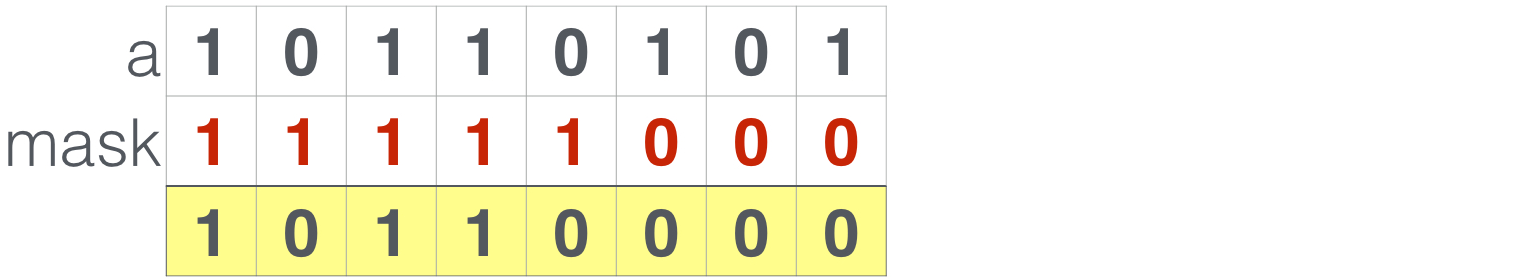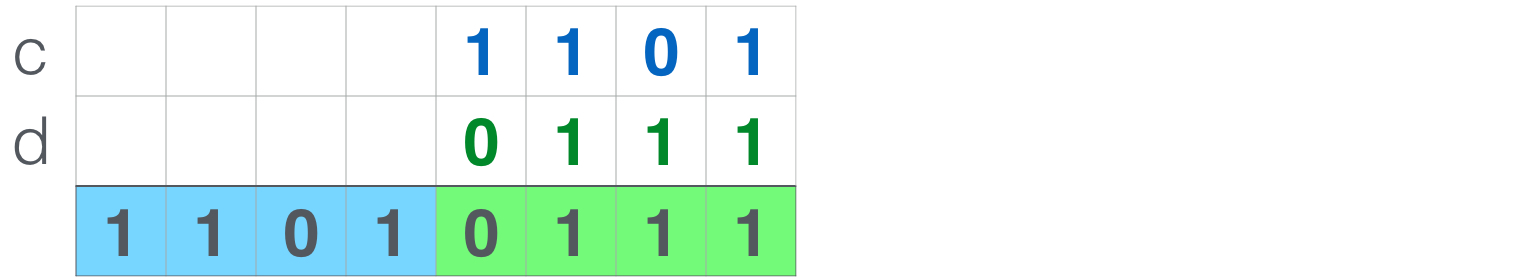# Solidity位操作运算符简介

https://www.interchains.cc/21790.html

Solidity位操作运算符简介是很好的区块链资料,他说明了区块链当中的经典原理，可以给我们提供资料,Solidity位操作运算符简介学习起来其实是很简单的，

### Solidity位操作运算符简介

• 智能合约
• Solidity

Solidity位操作运算有助于缩减以太坊eth交易的成本。本文介绍以太坊eth智能合约开发语言 Solidity中的位操作运算符，以及如何使用这些Solidity位操作符对合约数据执行位操作运算，例如与、或、非、异或等，同时也介绍如何实现Soldity不支持的取反、移位等操作。

## 1、Solidity位操作概述``bytes1 a = 0xb5; //     bytes1 b = 0x56; //  ``

## 2、与运算/AND`` a & b; // 结果: 0x14     ``

## 3、或运算/OR``a | b; // 结果: 0xf7    ``

## 4、异或运算/XOR``a ^ b; // 结果: 0xe3    ``

``0xe3 ^ a; // 结果: 0x56 == b    ``

## 5、非运算/NEGSolidity本身并不支持非运算，不过幸运的是你可以将变量与全1值 异或，就得到同样的结果：

`` a ^ 0xff; // Result: 0x4a     ``

## 6、移位运算/SHIFT

``00001230  ``

``01230000  ``

``00000123  ``

### 6.1 左移位``var n = 3;    var aInt = uint8(a); // Converting bytes1 into 8 bit integer   var shifted = aInt * 2 ** n;   bytes1(shifted);     // Back to bytes. Result: 0xa8     ``

### 6.2 右移位``var n = 2;    var aInt = uint8(a); // Converting bytes1 into 8 bit integer   var shifted = aInt / 2 ** n;   bytes1(shifted);     // Back to bytes. Result: 0x2d     ``

## 7、提取前N位``var n = 5;   var nOnes = bytes1(2 ** n - 1); // Creates 5 1s   var mask = shiftLeft(nOnes, 8 - n); // Shift left by 3 positions   a & mask; // Result: 0xb0    ``

## 8、提取后N位

``var n = 5;   var lastBits = uint8(a) % 2 ** n;   bytes1(lastBits); // Result: 0x15    ``

## 9、数据压缩``bytes1 c = 0x0d;   bytes1 d = 0x07;   var result = shiftLeft(c, 4) | d; // 0xd7   ``

## 完整源代码

`` contract BitsAndPieces {    function and(bytes1 a, bytes1 b) returns (bytes1) {    return a & b;    }     function or(bytes1 a, bytes1 b) returns (bytes1) {      return a | b;    }     function xor(bytes1 a, bytes1 b) returns (bytes1) {      return a ^ b;    }     function negate(bytes1 a) returns (bytes1) {    return a ^ allOnes();    }     function shiftLeft(bytes1 a, uint8 n) returns (bytes1) {      var shifted = uint8(a) * 2 ** n;      return bytes1(shifted);    }     function shiftRight(bytes1 a, uint8 n) returns (bytes1) {      var shifted = uint8(a) / 2 ** n;      return bytes1(shifted);    }     function getFirstN(bytes1 a, uint8 n) returns (bytes1) {      var nOnes = bytes1(2 ** n - 1);      var mask = shiftLeft(nOnes, 8 - n); // Total 8 bits       return a & mask;    }      function getLastN(bytes1 a, uint8 n) returns (bytes1) {    var lastN = uint8(a) % 2 ** n;    return bytes1(lastN);    }      // Sets all bits to 1     function allOnes() returns (bytes1) {    return bytes1(-1); // 0 - 1, since data type is unsigned, this results in all 1s.    }     // Get bit value at position    function getBit(bytes1 a, uint8 n) returns (bool) {    return a & shiftLeft(0x01, n) != 0;    }     // Set bit value at position    function setBit(bytes1 a, uint8 n) returns (bytes1) {    return a | shiftLeft(0x01, n);    }     // Set the bit into state "false"    function clearBit(bytes1 a, uint8 n) returns (bytes1) {    bytes1 mask = negate(shiftLeft(0x01, n));    return a & mask;    }   }  ``